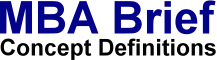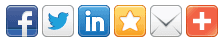# Bayesian Theory

 Definition: Bayesian Theory is a theory which is used by scientists to explain and predict decision-making. Bayes developed rules for weighing the likelihood of different events and their expected outcomes. According to Bayesian theory, managers should make decisions, based on a calculation of the probabilities of all the possible outcomes of a situation. By weighing the value of each outcome by the probability and summing the totals, they can calculate "expected values" for a decision. If the expected value is positive, then the decision should be accepted; if it is negative, it should be avoided. Does not include appreciation of downside risk nor unknown risks (see Plausibility Theory).
 Learn more about bayesian theoryMore on individual decision making: Anchoring Bias, Black Swan Theory, Bounded Rationality, Cognitive Bias, Cognitive Dissonance, more...

MBA Brief offers accurate and concise definitions of MBA concepts, frameworks, methods and models.